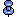# Step 1: Calculate the data row size

Rows that store variable-length data require more overhead than rows that contain only fixed-length data, so there are two separate formulas for computing the size of a data row.

## Fixed-length columns only

Use this formula if the table contains only fixed-length columns defined as NOT NULL:

 6 (Overhead) + Sum of bytes in all fixed-length columns Data row sizeData-only locked tables must allow room for each row to store a 6-byte forwarded row ID. If a data-only-locked table has rows shorter than 10 bytes, each row is padded to 10 bytes when it is inserted. This affects only data pages, and not indexes, and does not affect allpages-locked tables.

## Some variable-length columns

Use this formula if the table contains variable-length columns or columns that allow null values:

Formula

Example

8

8

+

Sum of bytes in all fixed-length columns

+

100

+

Sum of bytes in all variable-length columns

+

50

+

Number of variable-length columns * 2

+

4

Data row size

162